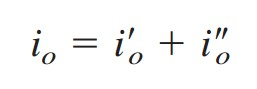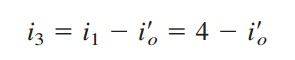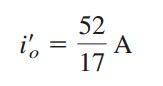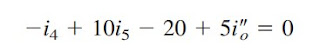omG8HI9LSKFW6lhCj8prTs0Z6lUhdOT9Jhi1Sf4m

# Superposition Theorem

If a circuit has two or more independent sources, one way to determine the value of a spesific variable (voltage or current) is to use nodal or mesh analysis like has been discussed in before. Another way is to determine the contribution of each independent source to the variable and then add them up. The latter approach is known as the superposition.

This circuit analysis theorems are classified as:

1. Superposition theorem
2. Source transformation
3. Thevenin theorem
4. Norton theorem
5. Maximum power transfer

## Superposition Theory

The idea of superposition rests on the linearity property.
The superposition principle states that the voltage across (or currents through) an element in a linear circuit is the algebraic sum of the voltages across (or currents through) that element due to each independent source acting alone.
The principle of superposition helps us to analyze a linear circuit with more than one independent source by calculating the contribution of each independent source separately. However, to apply the superposition principle, we must keep two things in mind:
1. We consider one independent source at a time while all other independent sources are turned off. This implies that we replace every voltage source by 0 V (or a short circuit), and every current source by 0 A (or an open circuit). This way we obtain a simpler and more manageable circuit.
2. Dependent sources are left intact because they are controlled by circuit variables.
With these in mind, we apply the superposition principle in three steps:
Steps to Apply Superposition Principle:
1. Turn off all independent sources except one source. Find the output (voltage or current) due to that active source using the techniques in previous explanation.
2. Repeat step 1 for each of the other independent sources.
3. Find the total contribution by adding algebraically all the contributions due to the independent sources.
Analyzing a circuit using superposition has one major disadvantage: It may very likely invole more work. If the circuit has three independent sources, we may have to analyze three simpler circuits each providing the contribution due to the respective individual source. However, superposition does help reduce a complex circuit to simpler circuits through replacement of voltage sources by short circuits and of current sources by open circuits.
Superposition theorem also can be used for ac circuit. Make sure to read it to advance your study.

## Superposition Examples

For better understanding let us review examples below:
1.Use the superposition theorem to find v in the circuit of Figure.(1)Figure 1
Solution :
Since there are two sources, let
where v1 and v2 are the contributions due to the 6 V voltage source and the 3 A current source, respectively. To obtain v1, we set the current source to zero, as shown in Fig.2(a).Figure 2
Applying KVL to the loop in Figure.(2a) gives
Thus,
We may also use voltage division to get v1 by writing
To get v2, we set the voltage source to zero, as in Figure.(2b). Using current division,
Hence,
And we find

2.Find io in the circuit of Figure.(3) using superposition.
The circuit in Figure.(3) involves a dependent source, which intact. We let(1)
where i'o and ino are due to the 4 A current source and 20 V voltage source respectively. To obtain i'o, we turn off 20 V source so that we have the circuit Figure.(4a). We apply mesh analysis in order to obtain i'o. For loop 1,(5)
Substituting (2) and (5) into (3) and (4) gives two simultaneous equations(8)
To obtain ino, we turn off the 4 A current source so that the circuit becomes that shown in Figure.(3b). For loop 4, KVL gives(10)
But i5 = -ino. Substituting this in (9) and (10) gives
Related Posts
SHARE